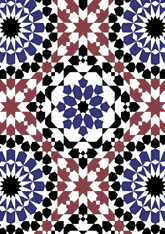data190/MOR0406

## Geometry

• The symmetry group of the tiling is *442 (p4m).
• All the internal angles of the constituent polygons are a multiple of 7.5°.
• Contains four squares.
• Contains four regular two-pointed star polygons with vertex angle of 60°.
• Contains two regular 8-pointed star polygons with vertex angle of 90°.
• Contains one regular 8-pointed star polygon with vertex angle of 45°.
• Contains one regular 16-pointed star polygon with vertex angle of 45°.
• There are 18 non-regular reflective tiles (including 2 kites and 2 darts) and one reflective pair.
• The tiling satisfies the two-colour condition.
• The tiling is edge-to-edge.
• As drawn, contains about 972 polygons.

## References

Publications referenced: